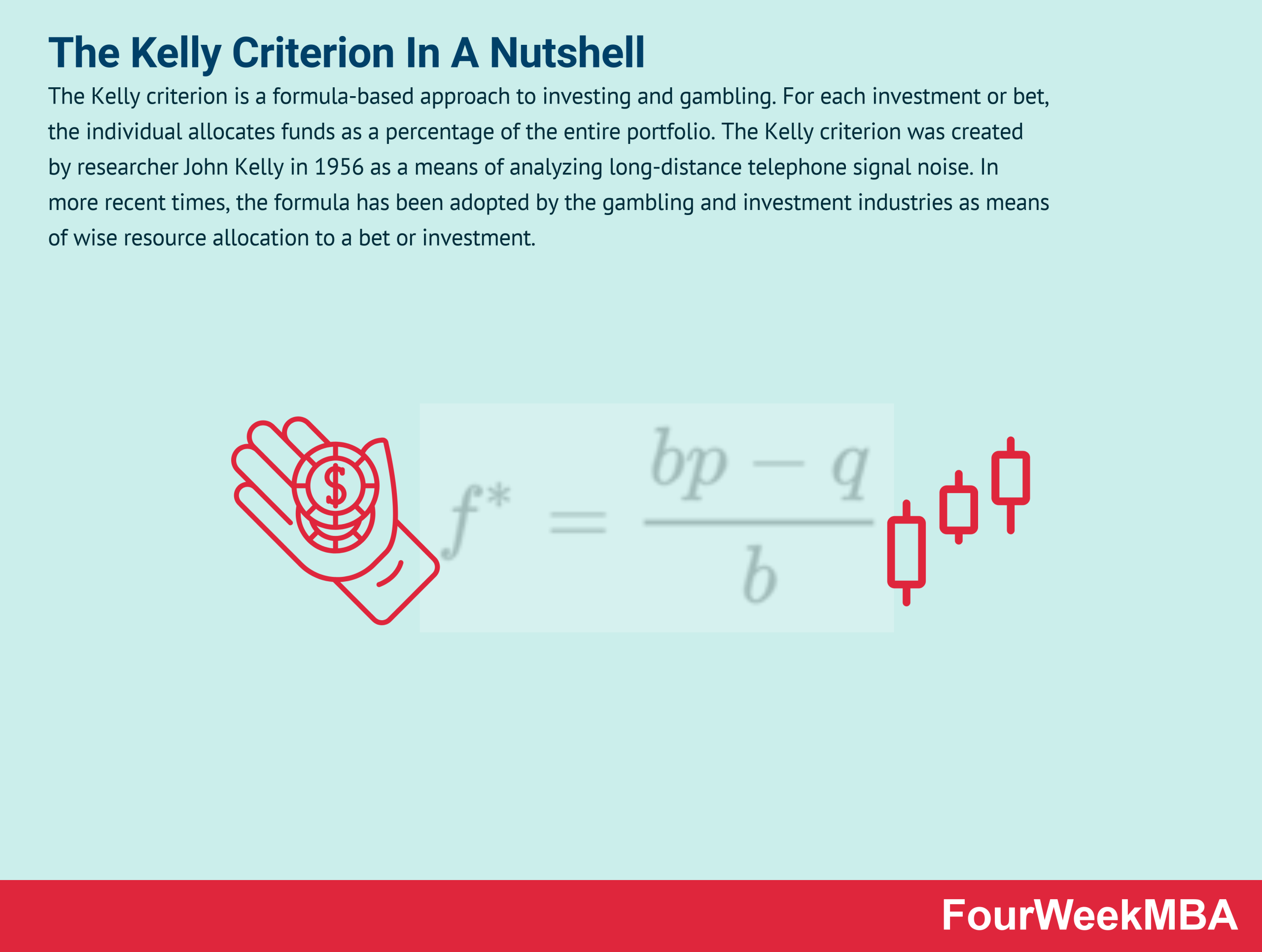# What Is The Kelly Criterion And Why It Matters In Business

The Kelly criterion is a formula-based approach to investing and gambling. For each investment or bet, the individual allocates funds as a percentage of the entire portfolio. The Kelly criterion was created by researcher John Kelly in 1956 as a means of analyzing long-distance telephone signal noise. In more recent times, the formula has been adopted by the gambling and investment industries as means of wise resource allocation to a bet or investment.

## For example:

• A blackjack player determining how much of their bankroll to wager on the next hand.
• A stock investor deciding on the percentage of their portfolio that should be devoted to speculative resource stocks.
• A real-estate investor questioning how much of their portfolio to devote toward condominiums in Miami Beach.

In each example, the goal of the gambler or investor is to grow their capital.

According to the Kelly criterion, the amount of money invested should be proportional to the knowledge of the bet or investment itself.

## Key components of the Kelly criterion formula

The Kelly criterion is expressed by the formula:

Here:

• f* = the total percentage of wealth that should be risked.
• p = the historical probability of a win. Ideally, the p-value needs to be above 0.50 or 50%.
• b = the decimal odds minus 1, otherwise known as the amount that could potentially be won or lost. For investors, calculate the b value by dividing the total number of trades yielding a positive amount by the total number of trades.
• q = the probability of failure (i.e. 1-p).

When interpreting the f* value, multiply it by 100 to get the percentage of total funds that should be risked. For example, if f* = 0.17 then the total percentage of funds allocated should be 17%.

## Kelly criterion limitations

Earlier, it was stated that knowledge of the bet was proportional to the amount of money invested in that bet.

This begs the question: how is knowledge obtained and what does it constitute?

The Kelly criterion defines knowledge as the perceived edge, itself defined as a betting advantage gained from exploiting bookmaker margins or possessing proprietary knowledge.

However, it is important to note that the formula is not foolproof. While it does define the point of maximum portfolio growth, the f* value is calculated from real-world probabilities that are best estimates only. Invariably, factors that influence the probability of winning are complex, obscured, or hard to define.

To reduce variance, many choose to estimate returns that are 30 to 50% of the calculated f* value. This is known as a safety margin, where risks are assumed to be higher than stated.

Other limitations of the Kelly criterion include:

• A focus on growth stocks. As growth stock profits are continually re-invested to encourage exponential growth, Kelly’s formula favors growth stock investors. Investors wishing to make smaller, more consistent income-stock profits may find the model too aggressive.
• Higher margin for error. Given that the f* value is the point of maximum potential growth, a figure only slightly higher brings substantially more risk, variance, and decreased profit. This means that undisciplined gamblers or investors prone to greed could suffer larger losses by ignoring the stated f* value.

## Key takeaways

• The Kelly criterion is a mathematical formula that guides gambling and investment decisions by way of risk-managed resource allocation.
• The Kelly criterion argues that the background knowledge of an investment or bet is directly proportional to the amount of money that should be allocated.
• While useful in a variety of scenarios, the Kelly criterion is nevertheless based on mostly unquantifiable probabilities that increase variance. As a result, a conservative approach to calculating investment percentages should be taken.